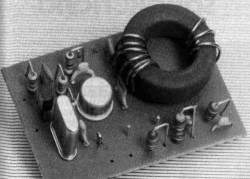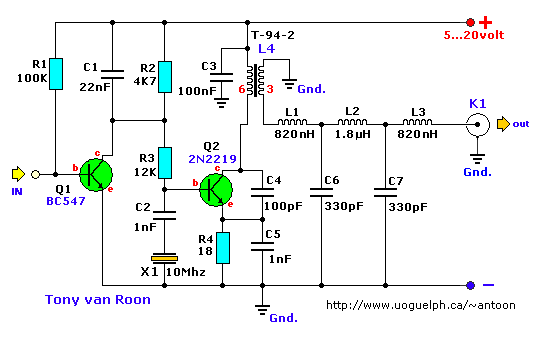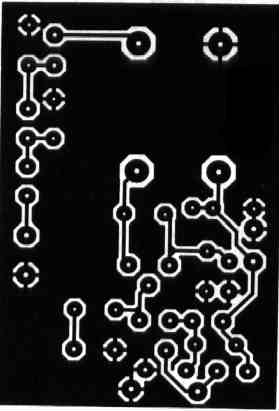30-Meter QRP (CW)
by P. Wyns, ON7WP (Belgium)

This transmitters' intended purpose is for morse-code only in the 30 meter band (10Mhz). It is a low-power QRP type and needs to be connected to your existing tranceiver. The harmonic rejections on the prototype were measured at 40dB on 20Mhz and 50dB on 30Mhz.Component Layout.1-5/16" x 2" (34mm x 52mm) double-sided.

Parts List
```  R1 = 100K       C1 = 22nF   L1,L3 = 820nH            BNC connector
R2 = 4K7     C2,C5 = 1nF       L2 = 1.8µH            Coolrib for Q2
R3 = 12K        C3 = 100nF     L4 = T-94-2 (Amidon)  Morse-key, single pole
R4 = 18 Ohm     C4 = 100pF     Q1 = BC547            All-metal case
xtal = 10Mhz   C6,C7 = 330pF     Q2 = 2N2219(A)```